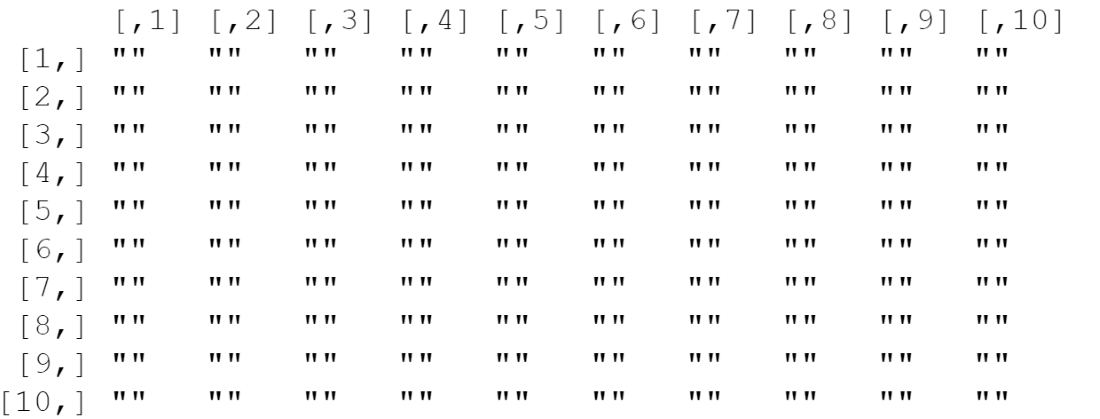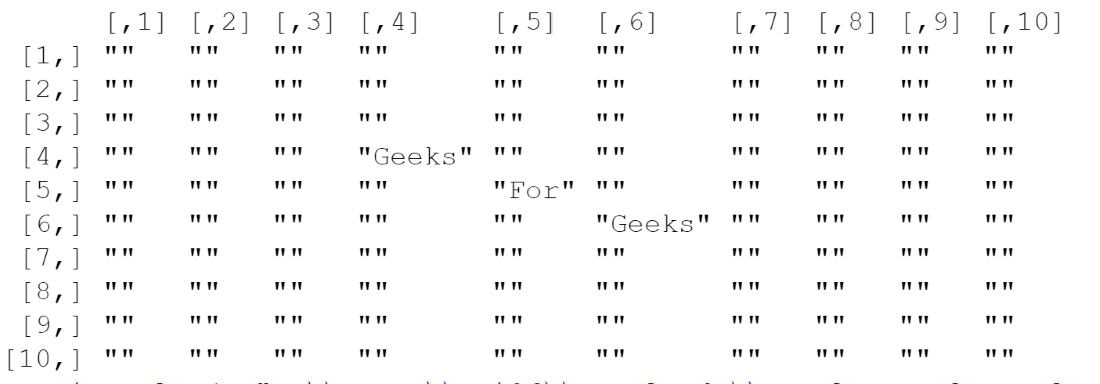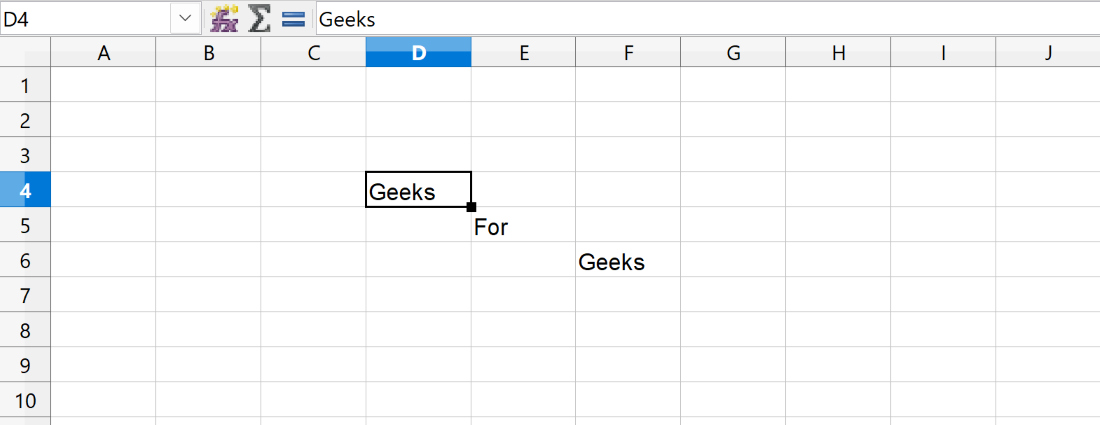Open in App
Not now

# How to write in a specific Row or column Using write.xlsx in R

• Last Updated : 21 Nov, 2022

The Excel File contains cells where the data can be stored in them. To deal with Excel Files in R programming Language we have a package named xlsx. Using the write.xlsx() function present in that package we are going to insert data into an excel file.

We are going to insert data at a specified row and column in R. So, As we know excel sheet is a Two-dimensional data set so we are going to first create a matrix with the required number of rows and columns later we are going to update the data at specified positions and simply insert that matrix into excel file.

Syntax: write.xlsx(df, file, sheetName, col.names, row.names, append, showNA, password)

• df – data frame or a 2D matrix object which is to be inserted into excel file
• file – the path to the output excel file is specified here
• col.names – logical value indicating if the column names of the data frame are to be written in the file
• row.names – logical value indicating if the row names of the data frame are to be written in the file

## Writing a matrix data to Excel using

Step 1: First, we need to install and load the required package(xlsx)

## R

 `install.packages``(``"xlsx"``)``library``(xlsx)`

Step 2: Next, we need to create an empty matrix with the required number of rows and columns here we will consider a 10 x 10 matrix.

## R

 `# Creation of Empty Matrix``m<-``matrix``(``''``, nrow=10, ncol=10)``m`

Output:Step 3: Later on we need to update the matrix where we want to insert data in an excel file. Let us consider if we want to insert data in the cell which is present at the 4th row and 4th column which is D4 then we have to update the matrix at the (4, 4) position of it.

## R

 `# Updating value present at fourth row and fourth column``m[4,4]<-``"Geeks"`` ` `m[5,5]<-``"For"``m[6,6]<-``"Geeks"``m`

Output:Step 4: Finally, we are going to insert this matrix into an excel file using the write.xlsx() function

## R

 `# Inserting matrix into an excel file using write.xlsx``xlsx::``write.xlsx``(m, ``                 ``"C:\\Users\\Downloads\\Sample_excel.xlsx"``,``                 ``col.names=``FALSE``,``                 ``row.names=``FALSE``)`

Output:My Personal Notes arrow_drop_up